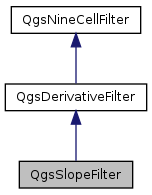QGIS API Documentation  2.0.1-Dufour
QgsSlopeFilter Class Reference

Calculates slope values in a window of 3x3 cells based on first order derivatives in x- and y- directions. More...

`#include <qgsslopefilter.h>`

Inheritance diagram for QgsSlopeFilter:[legend]
Collaboration diagram for QgsSlopeFilter:[legend]

## Public Member Functions

QgsSlopeFilter (const QString &inputFile, const QString &outputFile, const QString &outputFormat)
~QgsSlopeFilter ()
float processNineCellWindow (float *x11, float *x21, float *x31, float *x12, float *x22, float *x32, float *x13, float *x23, float *x33)
Calculates output value from nine input values.Public Member Functions inherited from QgsDerivativeFilter
QgsDerivativeFilter (const QString &inputFile, const QString &outputFile, const QString &outputFormat)
virtual ~QgsDerivativeFilter ()Public Member Functions inherited from QgsNineCellFilter
QgsNineCellFilter (const QString &inputFile, const QString &outputFile, const QString &outputFormat)
Constructor that takes input file, output file and output format (GDAL string)
virtual ~QgsNineCellFilter ()
int processRaster (QProgressDialog *p)
Starts the calculation, reads from mInputFile and stores the result in mOutputFile.
double cellSizeX () const
void setCellSizeX (double size)
double cellSizeY () const
void setCellSizeY (double size)
double zFactor () const
void setZFactor (double factor)
double inputNodataValue () const
void setInputNodataValue (double value)
double outputNodataValue () const
void setOutputNodataValue (double value)Protected Member Functions inherited from QgsDerivativeFilter
float calcFirstDerX (float *x11, float *x21, float *x31, float *x12, float *x22, float *x32, float *x13, float *x23, float *x33)
Calculates the first order derivative in x-direction according to Horn (1981)
float calcFirstDerY (float *x11, float *x21, float *x31, float *x12, float *x22, float *x32, float *x13, float *x23, float *x33)
Calculates the first order derivative in y-direction according to Horn (1981)Protected Attributes inherited from QgsNineCellFilter
QString mInputFile
QString mOutputFile
QString mOutputFormat
double mCellSizeX
double mCellSizeY
float mInputNodataValue
The nodata value of the input layer.
float mOutputNodataValue
The nodata value of the output layer.
double mZFactor
Scale factor for z-value if x-/y- units are different to z-units (111120 for degree->meters and 370400 for degree->feet)

## Detailed Description

Calculates slope values in a window of 3x3 cells based on first order derivatives in x- and y- directions.

Definition at line 24 of file qgsslopefilter.h.

## Constructor & Destructor Documentation

 QgsSlopeFilter::QgsSlopeFilter ( const QString & inputFile, const QString & outputFile, const QString & outputFormat )

Definition at line 20 of file qgsslopefilter.cpp.

 QgsSlopeFilter::~QgsSlopeFilter ( )

Definition at line 26 of file qgsslopefilter.cpp.

## Member Function Documentation

 float QgsSlopeFilter::processNineCellWindow ( float * x11, float * x21, float * x31, float * x12, float * x22, float * x32, float * x13, float * x23, float * x33 )
virtual

Calculates output value from nine input values.

The input values and the output value can be equal to the nodata value if not present or outside of the border. Must be implemented by subclasses

Implements QgsDerivativeFilter.

Definition at line 31 of file qgsslopefilter.cpp.

Referenced by QgsRelief::processNineCellWindow().

The documentation for this class was generated from the following files: